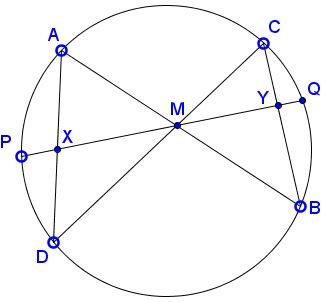# A proof of the butterfly theorem using the scale factor between the two wings.

### Theorem (The Butterfly Theorem)

Let $M\;$ be the midpoint of a chord $PQ\;$ of a circle, through which two other chords $AB\;$ and $CD\;$ are drawn. Let us assume that $A\;$ and $D\;$ do not belong to a same half-plane defined by $PQ.\;$ Let $X\;$ (respectively $Y)\;$ be the intersection of $AD\;$ (respectively $BC)\;$ and $PQ.$Then $M\;$ is also the midpoint of $XY.$

Let $O\;$ be the center of the circle. The points $A\;$ and $C\;$ belong to a same half-plane defined by $PQ,\;$ the points $B\;$ and $D\;$ to the other half-plane. For sake of simplicity, we can assume that $O\;$ belongs to the same half-plane as $B\;$ and $D.$

In the proof, we directly show that the ratio $\displaystyle\frac{\sin(CYM)}{\sin(AXM)}\;$ is nothing but the scale factor between the two wings $AMD\;$ and $CMB,\;$ which are similar by the Inscribed Angle Theorem:

$\displaystyle\frac{\sin(CYM)}{\sin(AXM)}=\alpha,\;$ where $\displaystyle\alpha=\frac{CM}{AM}=\frac{BM}{DM}=\frac{CB}{AD}.$

More precisely, we have:

$\displaystyle\sin(AXM)=\frac{DM^2-AM^2}{AD\cdot OM}.$

As a matter of fact:

\displaystyle\begin{align} DM^2-AM^2 &= ||\overrightarrow{DO}+\overrightarrow{OM}||^2-||\overrightarrow{AO}+\overrightarrow{OM}||^2\\ &=OD^2+OM^2+2\overrightarrow{DO}\cdot\overrightarrow{OM}-(OA^2+OM^2+2\overrightarrow{AO}\cdot\overrightarrow{OM})\\ &=2\overrightarrow{DO}\cdot\overrightarrow{OM}\; \text{since }OA=OD\\ &=2AD\cdot OM\sin(AXM)\;\text{as } OMX=OMY=\frac{MX+OMY}{2}=\frac{\pi}{2}, \end{align}

because triangle $OMP\;$ and $OMQ\;$ are congruent. Similarly, we have:

$\displaystyle\sin(CYM)=\frac{BM^2-CM^2}{CB\cdot OM}.$

Thus:

\displaystyle\begin{align} \frac{XM}{YM}&=\frac{AM}{CM}\cdot\frac{CM}{YM}\cdot\frac{XM}{AM}\\ &=\frac{AM}{CM}\cdot\frac{\sin(CYM)}{\sin(YCM)}\cdot\frac{\sin(XAM)}{\sin(AXM)} \end{align}

by the law of sines, applied in triangles $AXM\;$ and $CYM.\;$ Now we continue using the Inscribed Angle Theorem, as the next step:

\displaystyle\begin{align} \frac{XM}{YM}&=\frac{AM}{CM}\cdot\frac{CM}{YM}\cdot\frac{XM}{AM}\\ &=\frac{AM}{CM}\cdot\frac{\sin(CYM)}{\sin(AXM)}\\ &=\frac{AM}{CM}\cdot\frac{BM^-CM^2}{CB\cdot OM}\cdot\frac{AD\cdot OM}{DM^2-AM^2}\\ &=\frac{AM}{CM}\cdot\frac{AD}{CB}\cdot\frac{BM^2-CM^2}{DM^2-AM2}\\ &=\frac{1}{\alpha}\cdot\frac{1}{\alpha}\cdot\alpha^2\\ &=1. \end{align}### Butterfly Theorem and Variants